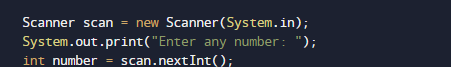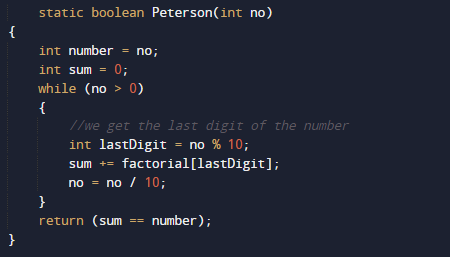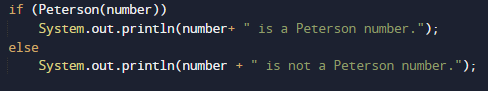# Program to check Peterson Number in Java

In this tutorial you will learn about the Program to check Peterson Number in Java and its application with practical example.

In this tutorial, we will learn to create a Java Program to check Peterson Numbers in Java using Java programming.

## Prerequisites

Before starting with this tutorial we assume that you are best aware of the following Java programming topics:

• Java Operators.
• Basic Input and Output function in Java.
• Class and Object in Java.
• Basic Java programming.
• If-else statements in Java.
• For loop in Java.

## What is Peterson Number in Java

A number is called Peterson Number if  sum of its factorials of each digit is equal to the sum of the number itself.

145 = 5! + 4! + 1!
145 = 120 + 24 +1
145 = 145

Explanation: factorial of
!5 = 5*4*3*2*1=120.
!4=4*3*2*1=24.
!1=1.
So sum of its factorial gives the number itself called Peterson.

## Program to check Peterson Number in Java.

In this our program we will find given number is a Peterson number or not in using java. We would first declared and initialized the required variables. Next, we would prompt user to input the a number.Later we  find number is  Peterson number or not let’s have a look at the code.

## Output

Peterson NumberNot a Peterson numberIn the above program, we have first declared and initialized a set variables required in the program.

• number= it will hold entered number.
• sum= it will hold sum of digit
• no = it will also hold value of number
• lastdigit= last digit of a number.

After declaring variables in the next statement user will be prompted to enter a value and  which will be assigned to variable ‘number’.And after that we check condition for Peterson number if condition get satisfied it means the given number is Peterson number if not then it is not  Peterson number.

### Logic Behind of Finding Peterson Number.

• First we take and initialize a number  to (number).
• Find the last digit (last digit) of the given number by user.
• Find the factorial of all the digits.
• Add the factorial of all digits to (sum) variable as shown in image below.
•• Repeat steps until find all the factors of given number.
• Compare sum with number if( sum==number). If they are equal, the given number is Peterson,• else not.

In this tutorial we have learn about the Program to check Peterson Number in Java and its application with practical example. I hope you will like this tutorial.